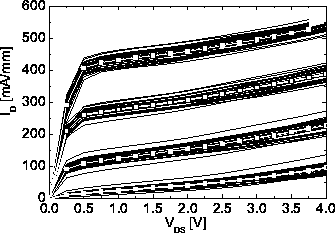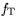Next: 5.1.1 Mathematical Background and Up: 5. Statistical Characterization of Previous: 5. Statistical Characterization of

# 5.1 Statistical Analysis of III-V HEMT Data

Semiconductor circuit and device simulation very much relies on the reproducibility of semiconductor processes. Fig. 5.1 show output curves fields obtained from one wafer. A mean device is extracted as the mean value of the drain current for each bias applied.Such a set of mean values of devices, which is further referred to as mean devices, can be determined for wafers, wafer runs, or even for whole technologies in production. Systematic shifts and long term production deviations can be calculated, e.g. in order to evaluate the feasibility of an optimization.

III-V device data used for comparison with or even calibration of device simulator results require statistical analysis of data. Statistical principles can be used to decide whether suggested device modifications can be called optimization, i.e. whether parameters such as yield or sensitivity towards critical parameters are affected. In the following a statistical extraction approach for device parameters is applied to demonstrate the interaction between the global simulation parameter extraction procedure described for device simulation in Section 3.6 and the acquisition of measured data for comparison. This approach is primarily useful since for III-V technology simulation data of the underlying process steps are not available, that could be used to center or optimize devices in a more complete simulation approach, as presented for MOSFETs in [219,277].

A wafer mapping measurement result normally determines the mean and variance values of several quantities observed. Hardly ever the variance values of the quantities are analyzed, e.g. by covariance analysis. The covariance of the processed wafer contains a high amount of information. A more intuitive approach to covariance analysis is highlighted in the following example: A positive shift of the threshold voltages and a rise of thevalue will lead to the conclusion that the gate-to-channel separation has statistically diminished for the observed wafer. It remains mostly undetermined whether this analysis is really significant in a statistical sense.

A method used for the analysis of the covariance of wafer map data is factor analysis or, as a special case, principal component analysis (PCA). Principal component analysis is a formalization of a method that is almost "naturally" applied by an educated reader of wafer map results to reduce complexity. PCA was demonstrated in a similar approach by Krupenin et al. in  to evaluate DC data taken from 3 inch InP HEMT wafers. PCA is only one of the possible ways to analyze and simplify the multivariate data set. A more generalized method to analyze the correlation matrix is factor analysis. These methods are discussed hereafter, as a device simulator generically is able to evaluate physical sources of covariance suitable for data interpretation. The numerical calculations are performed by the commercially available statistic program SPSS V 10.0 .

SubsectionsNext: 5.1.1 Mathematical Background and Up: 5. Statistical Characterization of Previous: 5. Statistical Characterization of
Quay
2001-12-21